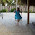As a fifth and sixth grade teacher, I know the struggle is R-E-A-L for our kiddos to learn about fractions!  This is evidenced by the sheer volume of time dedicated to this concept compared to other mathematical concepts.  As if adding and subtracting fractions weren’t difficult enough, now our fourth, fifth and sixth graders are being asked to multiply and divide fractions!
It may be tempting to skip right to the algorithm and skip the conceptual understanding, but I would highly discourage this.  The conceptual understanding is what will stick with the child and help them apply this concept to more complex situations and for your students who require interventions, this will help them tremendously.  Here are a couple of quick ways to introduce division of fractions in a more concrete way.

Dividing Whole Number By Fraction

How many ____ are in ____?
fraction    whole

Ready, Set, Break!
1. Ready: The whole class stands up.
2. Set: Huddle as a class (come towards the middle of the room).
3. Break: Then “break” off into the called out fraction and the groups then determine how many people are in their group and yells out the number of students.

Ex.  30 students in class huddle.  Teacher (or student caller) calls out the Break Number ½ .  The class then breaks into two even groups and shouts out the number of students in each ½ ...15! How many ½’s are in 30?

Ex. 28 students in class huddle.  Teacher calls out the Break Number ¼ . The class then breaks into four equal groups and shouts out the number of students in each group...7! How many ¼ 's are in 28?

Ex. 33 students in class huddle.  Teacher calls out Break Number ⅓ .  The class then breaks into four equal groups and shouts out the number of students in each group...11! How many ⅓’s are in 33?

Dividing Fraction by Fraction

How many groups of _____ are in _____?
fraction         fraction

Kelly made brownies last night.  She has ¾ of the pan left.  She wants to divide her leftovers into ⅛ slice servings.  How many servings will she have?

I find that introducing a new math concept with a real world problem helps them to better come up with ways to solve it because they don't necessarily already know the algorithm. Watch the video I made below to see how this is solved using a pan of brownies. Brownies in class...always a hit and sure to have your students on the edge of their seat. The real question is, will there be enough for each student to have a piece? Feel free to use this video in your class, but actually doing this problem with your students will be more engaging and memorable.

Dividing Mixed Number by Fraction

How many groups of _____ are in ____?
fraction       mixed numberThis website shows division of fractions by visually dividing a number line.  It breaks it down step-by-step, is color-coded, and can be assigned during intervention or for homework as extra practice. I like that it shows each step with a model and also the algorithm at the bottom.

How do you engage your students in conceptual understanding of tough concepts like division of fractions?  Let me know what you think of these strategies and how you teach this by commenting below.

Not on division of fractions yet?  Check out my resource on Multiplying Fractions while it is on sale through the end of this weekend!by Lisa Prins

#### 3 comments

1.I love how you show how to divide fractions in a concrete way! I will definitely be using the class huddle and brownie ideas when we get to that unit!!

1.Yay! I'm so glad you found this helpful!

2.What website is the 2nd picture from with the bar models?